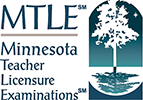## Test Framework Worksheet

Check this box if the course listed above covers the content described by this objective
Check this box if the course listed above covers the content described by this objective
Check this box if the course listed above covers the content described by this objective
Check this box if the course listed above covers the content described by this objective
Check this box if the course listed above covers the content described by this objective
Check this box if the course listed above covers the content described by this objective
Check this box if the course listed above covers the content described by this objective
Check this box if the course listed above covers the content described by this objective
Check this box if the course listed above covers the content described by this objective
Check this box if the course listed above covers the content described by this objective
Check this box if the course listed above covers the content described by this objective
Check this box if the course listed above covers the content described by this objective
Check this box if the course listed above covers the content described by this objective
Check this box if the course listed above covers the content described by this objective
Check this box if the course listed above covers the content described by this objective
Check this box if the course listed above covers the content described by this objective
Check this box if the course listed above covers the content described by this objective
This worksheet allows users to map the test framework to the curriculum by entering up to five courses in the course name fields, and checking the boxes if the content of the course covers the information described by the test objectives in the rows below.
Subarea Objective Number Objective Description
Subtest 1: Test Code 064
Subarea I. Concepts in Physics
• Multiple-Choice Questions: 16
• Test Weight: 33%
0001 Understand the principles and procedures of scientific inquiry.
0002 Demonstrate knowledge of problem solving in physics and the relation of physics to everyday life.
0003 Understand the content and methods for developing students' content-area reading skills to support their reading and learning in physics.
Subarea II. Linear and Rotational Motion
• Multiple-Choice Questions: 23
• Test Weight: 45%
0004 Analyze motion in one and two dimensions.
0005 Analyze forces and systems of interacting objects.
0006 Understand the conservation of mechanical energy.
0007 Understand the conservation of momentum.
Subarea III. Simple Harmonic and Wave Motion
• Multiple-Choice Questions: 11
• Test Weight: 22%
0008 Understand simple harmonic motion.
0009 Understand waves and wave propagation.
Subtest 2: Test Code 065
Subarea I. Electricity and Magnetism
• Multiple-Choice Questions: 19
• Test Weight: 38%
0010 Analyze electric circuits.
0011 Demonstrate knowledge of electric and magnetic fields.
0012 Demonstrate knowledge of electromagnetic induction.
Subarea II. Geometrical and Physical Optics
• Multiple-Choice Questions: 12
• Test Weight: 25%
0013 Demonstrate knowledge of geometrical optics.
0014 Demonstrate knowledge of physical optics.
Subarea III. Kinetic Theory and Contemporary Physics
• Multiple-Choice Questions: 19
• Test Weight: 37%
0015 Demonstrate knowledge of thermodynamics and the kinetic theory of matter.
0016 Demonstrate knowledge of the fundamental principles of special relativity and atomic and subatomic physics.
0017 Demonstrate knowledge of the fundamental principles of quantum physics.

Print this worksheet to save for your records, or to fill out offline: